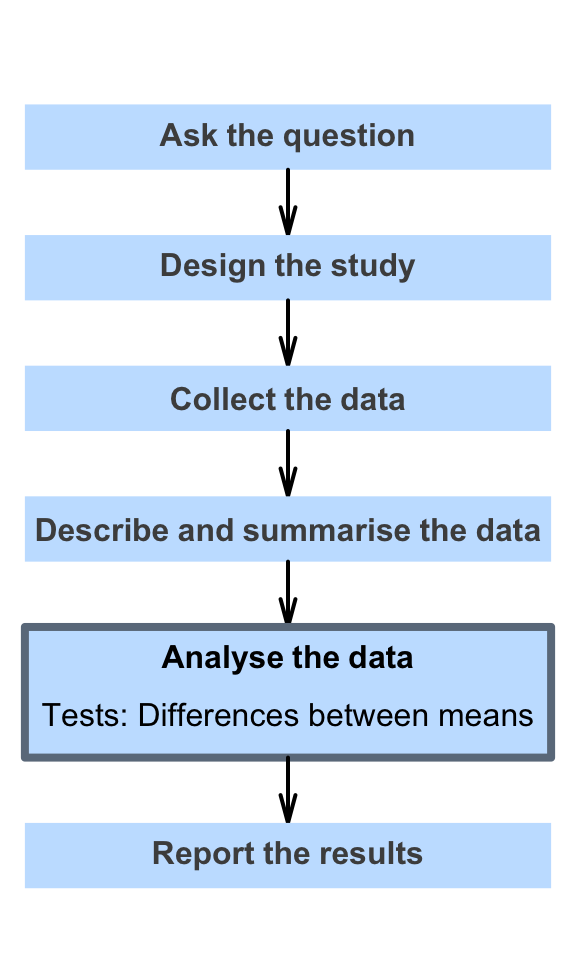# 30 Hypothesis tests for means of two independent groups

So far, you have learnt to ask a RQ, identify different ways of obtaining data, design the study, collect the data describe the data, summarise data graphically and numerically, understand the tools of inference, and to form confidence intervals.

In this chapter, you will learn about hypothesis tests for the difference between two means. You will learn to:

• conduct hypothesis tests for comparing two means.
• determine whether the conditions for using these methods apply in a given situation.